# Use different types of vector arrow in LaTeX(with example).

Vectors are represented in different ways. For this reason, it is best practice to use vector arrows responsibly according to the shape of the whole symbol.

You usually use the default \vec command for vector arrow symbols.

\documentclass{article}
\begin{document}
$$\vec{p}$$
$$\vec{\ddot{p}}$$
$$\vec{p_{q}}$$
$$\vec{pq}$$
$$\vec{pqr}$$
\end{document}

Output :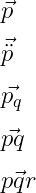You notice that the vector arrow above does not work responsibly with each of the above arguments except for the single character. So, this command is best used for arrow marking on a single character.

And the second default command is \overrightarrow. This command returns the size of the vector arrow according to the size of vector variable. For example

\documentclass{article}
\begin{document}
$$\overrightarrow{a}$$
$$\overrightarrow{ac}$$
$$\overrightarrow{p^{q}}$$
$$\overrightarrow{p_{q}}$$
$$\overrightarrow{AB}$$
\end{document}

Output :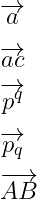Although the size of the vector arrow matches according to the length of the argument(vector variable). But, it is not right to use it in terms of style.

## Use esvect package for vector arrow

The most important command for vector arrow is the \vv of esvect package. Because there are different types of responsive arrows stored in this command.

\documentclass{article}
\begin{document}
$$\vec{p}$$
$$\vec{\ddot{p}}$$
$$\vec{p_{q}}$$
$$\vec{pq}$$
$$\vec{pqr}$$
\end{document}

Output :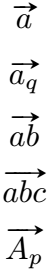If you look at the output above, the vector arrow is very nicely placed on top of each vector variable.

And for different styles of arrows, you have to pass different characters(a,b,..,g) as optional arguments with esvect package. But, the command will remain the same for each arrow. So, look at the table below.

 Optional Argument Command and symbol a \vv{abc}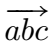b \vv{abc}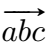c \vv{abc}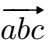d \vv{abc}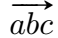e \vv{abc}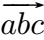f \vv{abc}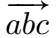g \vv{abc}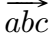h \vv{abc}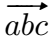For example, I will take the c optional argument from the table above. But, you can check with each optional argument.

\documentclass{article}
\usepackage[c]{esvect}
\begin{document}
$$\vv{r}$$
$$\vv{\ddot{r}}$$
$$|\vv{\ddot{r}}|$$
$$\frac{d(\vv{r})}{dt}$$
\end{document}

Output :And if you want to use subscripts and superscripts with vector variables, you can use the \va* command instead of the \va command.

\documentclass{article}
\usepackage{esvect}
\begin{document}
$$\vv*{p^{q}}$$
$$\vv*{p_{q}}$$
\end{document}

Output :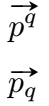## Another package for vector arrow

There are also some packages to denote the vector arrow. For example

### 1. Use physics package

Physics package contains the \vectorarrow command to denote the arrow symbol. Which is written in the form of \va.

\documentclass{article}
\usepackage{physics}
\begin{document}
$$\va{a}$$
$$\va{a_{q}}$$
$$\vectorarrow{ab}$$
\end{document}

Output :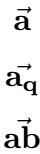And it is best to use this command in the case of a single character like \vec command.

### 2. Use mathabx package

mathabx package contains the \widearrow command to denote this symbol, and it is better to use this command than \overrightarrow.

\documentclass{article}
\usepackage{mathabx}
\begin{document}
$$\widearrow{\ddot{r}}$$
$$\widearrow{ab}$$
$$\widearrow{abc}$$
$$\widearrow{abcd}$$
\end{document}

Output :### 3. Use marvosym package

\Vectorarrow and \Vectorarrowhigh commands are similar to \vec commands. That is, you can use these commands only in the case of single character vector variables. And you don’t have to pass any arguments(vector variable).

\documentclass{article}
\usepackage{marvosym}
\begin{document}
r\Vectorarrow  \\
p\Vectorarrowhigh
\end{document}

Output :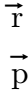In this tutorial, many packages and commands for representing vector arrow symbols are discussed in depth. In my opinion, the best practice is to use esvect package and vv command for the structure and beauty of this symbol.